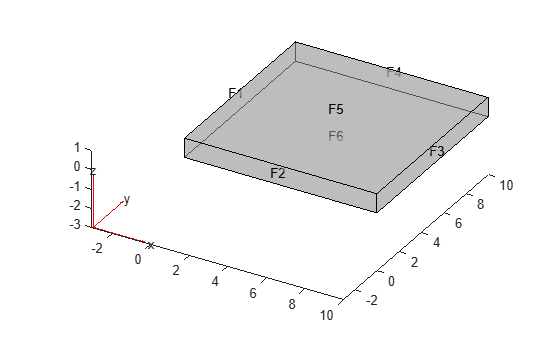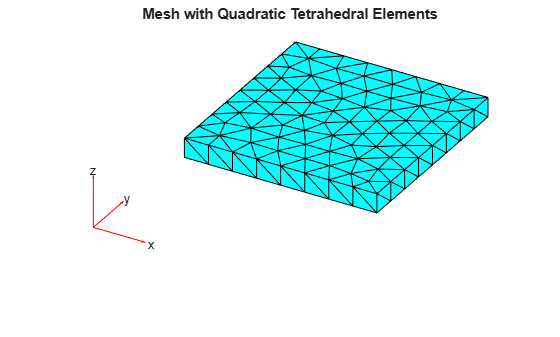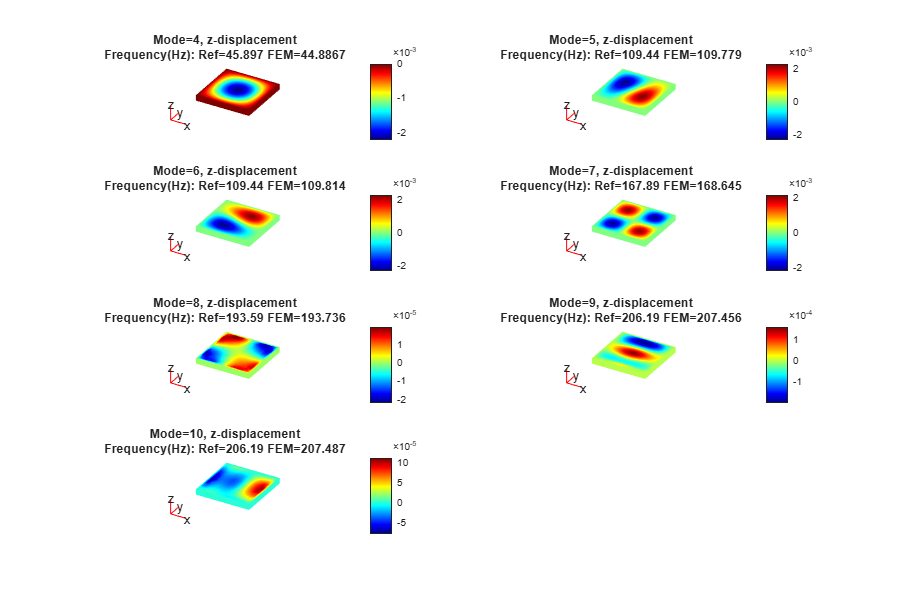Documentation

Vibration of Square Plate

This example shows how to calculate the vibration modes and frequencies of a 3-D simply supported, square, elastic plate.

The dimensions and material properties of the plate are taken from a standard finite element benchmark problem published by NAFEMS, FV52 (See Reference).

First, create a structural model container for your 3-D modal analysis problem. This is a container that holds the geometry, properties of the material, body loads, boundary loads, boundary constraints, and mesh.

model = createpde('structural','modal-solid');

Import an STL file of a simple plate model using the importGeometry function. This function reconstructs the faces, edges, and vertices of the model. It can merge some faces and edges, so the numbers can differ from those of the parent CAD model.

importGeometry(model,'Plate10x10x1.stl');

Plot the geometry and turn on face labels. You need the face labels when defining the boundary conditions.

figure
hc = pdegplot(model,'FaceLabels','on');
hc(1).FaceAlpha = 0.5;
title('Plate with Face Labels')Define the elastic modulus of steel, Poisson's ratio, and the material density.

structuralProperties(model,'YoungsModulus',200e9, ...
'PoissonsRatio',0.3, ...
'MassDensity',8000);

In this example, the only boundary condition is the zero $z$-displacement on the four edge faces. These edge faces have labels 1 through 4.

structuralBC(model,'Face',1:4,'ZDisplacement',0);

Create and plot a mesh. Specify the target minimum edge length so that there is one row of elements per plate thickness.

generateMesh(model,'Hmin',1.3);
figure
pdeplot3D(model);
title('Mesh with Quadratic Tetrahedral Elements');For comparison with the published values, load the reference frequencies in Hz.

refFreqHz = [0 0 0 45.897 109.44 109.44 167.89 193.59 206.19 206.19];

Solve the problem for the specified frequency range. Define the upper limit as slightly larger than the highest reference frequency and the lower limit as slightly smaller than the lowest reference frequency.

maxFreq = 1.1*refFreqHz(end)*2*pi;
result = solve(model,'FrequencyRange',[-0.1 maxFreq]);

Calculate frequencies in Hz.

freqHz = result.NaturalFrequencies/(2*pi);

Compare the reference and computed frequencies (in Hz) for the lowest 10 modes. The lowest three mode shapes correspond to rigid-body motion of the plate. Their frequencies are close to zero.

tfreqHz = table(refFreqHz.',freqHz(1:10));
tfreqHz.Properties.VariableNames = {'Reference','Computed'};
disp(tfreqHz);
Reference     Computed
_________    __________

0      2.4285e-06
0      3.4344e-06
0      3.2672e-05
45.897          44.871
109.44          109.74
109.44          109.77
167.89          168.59
193.59          193.74
206.19          207.51
206.19          207.52

You see good agreement between the computed and published frequencies.

Plot the third component ($z$-component) of the solution for the seven lowest nonzero-frequency modes.

h = figure;
h.Position = [100,100,900,600];
numToPrint = min(length(freqHz),length(refFreqHz));
for i = 4:numToPrint
subplot(4,2,i-3);
pdeplot3D(model,'ColorMapData',result.ModeShapes.uz(:,i));
axis equal
title(sprintf(['Mode=%d, z-displacement\n', ...
'Frequency(Hz): Ref=%g FEM=%g'], ...
i,refFreqHz(i),freqHz(i)));
endReference

 National Agency for Finite Element Methods and Standards. The Standard NAFEMS Benchmarks. United Kingdom: NAFEMS, October 1990.

Partial Differential Equation Toolbox DocumentationGet trial now